# Top 15 Greatest Mathematicians and their Contribution to the World

Mathematics as a subject is the basis of progress of mankind as it holds a key position in scientific experiments, calculation, and discoveries. While many students dread this subject, it can be quite interesting when you think of it as a means to solve the mysteries of the universe. The history of the world has come across genius mathematicians, who have changed the world with their great contributions in this field.

Here is a list of top 15 greatest mathematicians, along with their contributions to the world:

### 15. John von NeumannJohn was born in 1903 and he lived until 1957. He was born in Budapest and he helped in designing the architecture of the computers. The modern computers are based on the architecture provided by him. This is because he helped in the inception of a series which forms the base of all the electronic devices. He was also a chemical engineer but he was always more interest in Math. Most of his discoveries were made around set theory, geometry, game theory, quantum mechanics and computer science. He was also an integral part of Manhattan project.

### 14. Pythagoras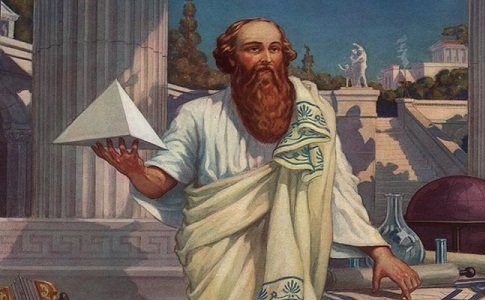Pythagoras of Samos was a Greek mathematician, scientist, and philosopher, who inked a new phase in Mathematics by bringing forth the famous Pythagoras Theorem, named after him to honor this genius. This Theorem continues to be the basis of numerous other theorems in Mathematics and Geometry and is of relevance in modern times too.

### 13. Wilhelm LeibnizNext on the list of mathematical prodigies is the German philosopher and mathematician, Wilhelm Leibniz, who played an instrumental role in the invention of calculus. Another contribution of this genius was that he had a major hand in the invention of mechanical calculators. He also contributed in the field of topology.

### 12. Isaac Newton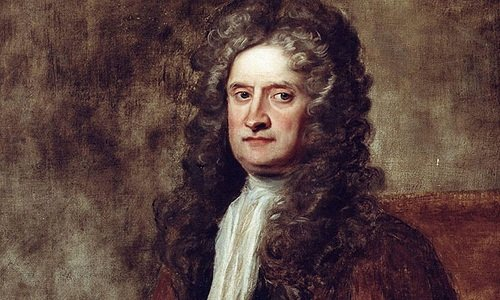The Englishman Isaac Newton is better known as a scientist who discovered the Law of Gravitation and the Three Laws of Motion, but he was an equally great mathematician too. He is credited with the formulation of Binomial Theorem and infinitesimal calculus, the latter one, along with Gottfried Leibniz.

### 11. Srinivasa Ramanujan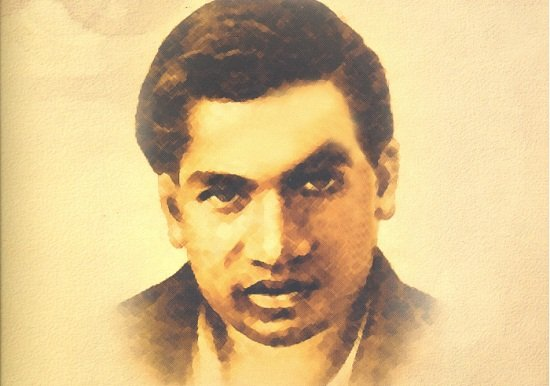This is another Indian mathematician in our list and he lived between 1887 and 1920. It is known that he never received a formal training in math and even after that, he made several contributions to mathematical analysis, number theory, continued fractions and infinite series. He is the man behind Hardy-Ramanujan Littlewood circle and he also worked on products of hypergeometric series.

### 10. Andrew Wiles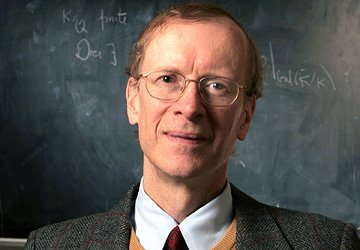In this list of greatest mathematicians of the world is the contemporary genius, Andrew Wiles, who has contributed to the field of modern mathematics with the proof of Fermat Last Theorem, which took him seven long years of hard work and dedication.

### 9. Leonardo Fibonacci BigolloLeonardo Fibonacci Bigollo was an acclaimed Italian mathematician, who is the inventor of the famous Fibonacci Series. Additionally, he was responsible for the introduction of Arabic number system in Europe. His contribution to modern mathematics can never be denied.

### 8. Bernhard RiemannThe next name on the list of top 10 greatest mathematicians in the world is that of the German scientist, Bernhard Riemann, who made great contributions in the field of number theory and differential geometry and analysis. He came from a poor family but was a brilliant mathematician, who is also credited with the introduction of Riemann Hypothesis, which deals with distribution of prime numbers.Bhaskar was another Indian scientist who made a lot of contribution is the field of mathematics. Bhaskar was born in Karnataka and he was born in 1114. He solved several astronomical problems and he is also known for his book called Siddhanta Siromani. One of his famous theory was that any number divided by 0 is infinity and the sum of any number and infinity is also infinity.

### 6. Carl Friedrich Gauss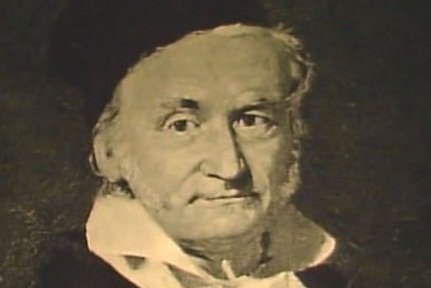This German mathematician was honored with the title of “Prince of Mathematicians” and his contributions in the mathematical field are diverse, ranging from algebra to statistics, number theory, differential geometry, and analysis. He exhibited extraordinary skills as a teenager and even wrote his magnum opus called Disquisitiones Arithmeticae when he was just 21 years old.

### 5. Alan TuringAlan was a British Mathematician and he is often addressed as the father of computer science. During the Second World War, he broke the Nazi crypto code and this helped the administration is protecting a lot of property and targets. It is also said that the allies won the world war because of Alan’s contribution. And it is also known that he was a gay and he was given a punishment to undergo a hormone treatment for the same. He later committed suicide

### 4. Aryabhata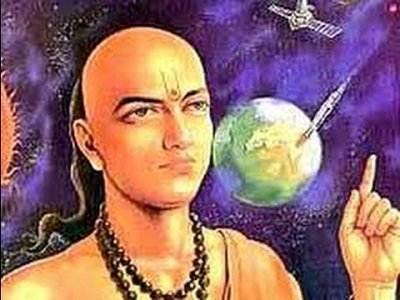Aryabhata is the great Indian mathematician from the ancient period who will always be revered for his glorious contribution to the field. He was the first one to introduce the place value system by denoting numbers with letters. He also discovered fats like the position of the planet, their revolution around the sun and measured the number of days in a year.

### 3. Euclid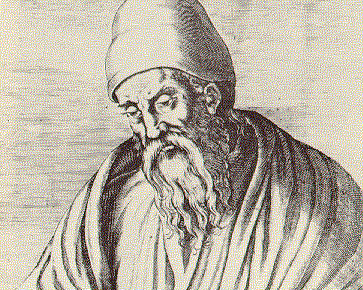Featuring next on the list of top 10 greatest mathematicians in the world history is Euclid, who is regarded as the Father of Geometry. He lived around 300 BC, but his great works on elements, geometry and number theory are used till date as a part of modern education in mathematics.

### 2. Euler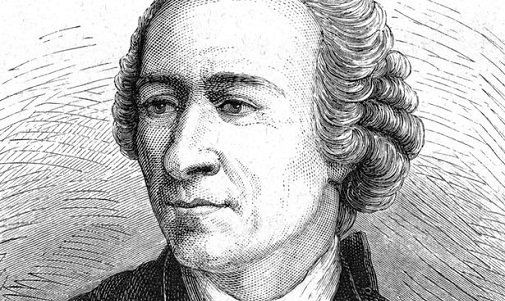The Swiss mathematician Euler is regarded as one of the greatest mathematical geniuses in the history of the world. His discoveries spanned the fields of calculus, geometry, algebra, graph theory and trigonometry. He also introduced the notions of mathematical and trigonometric function, which have become the framework of modern mathematics today.

### 1. BrahmaguptaBrahmagupta was a well-known Indian mathematician and astronomer. He was born in year 598 and he passed away in year 665. As per the records, it is known that he was the first person to establish rules about computing with 0. Most of his texts are in Sanskrit and he has also given the solution to the general linear equation. At last, he also gave the sum of the square and cubes of first n integers. Without him, we would not have been here as he formed the basis of modern mathematics.

Some other mathematicians who have been credited with some great contributions to the world are Rene Descartes, Alan Turing, and much more.